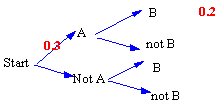### CFA Practice Question

There are 985 practice questions for this topic.

### CFA Practice Question

For the tree diagram shown with P(A) = 0.3 and P(B) = 0.6, which of the following is false?A. P(not A) = 0.7
B. Events A and B are dependent.
C. P(not B) = 0.4
D. Events A and B are independent.

We know events A and B are NOT independent events because P(B | A) = 2/3 and it was given that P(B) = 0.6. Because P(B | A) is not equal to P(B), the events are dependent.

User Comment
vincenthuang B is correct
Pooh How would you know p(not b) is 0.4? anyone?
jminard Vincent: B is not correct. It's false.
Pooh: it's given that P(B) = 0.4, so P(not B) is 1 - 0.4 = 0.6
Yooo jminard is incorrect in both instances. A and B are dependent. The outcome of A affects outcome of B. P(B) is not given. It is possible that the P(notB)= 0.4
Rotigga D is correct. P(A) = 0.3; P(B) = 0.6 . From the diagram, we get P(A and B) = 0.2, which does not equal P(A) * P(B) = 0.18; therefore, the two events are Dependent.
bobert A) True: P(not A) = .7
--> A is given as P(A)=.3 P(AS) + (PAS^c) = 1
Therefore after filling all in, we get .7
B) True: They are dependent because of the reason posted by Yooo and Rotigga
C) True: Same reason as in A). Do not be fooled by the .2 which is not P(B) it is the P(B|A).
D) False: Due to the opposite of B).
StanleyMo P (B) = 0.4, so P(not B) =0.6 :)
JKiro Pooh:
we are given that the P(B) = 0.6; the complement or the P(not B) = 0.4
jlevee none of this matters. look at the diagram - if they were independent, you'd have the same probability of reaching B regardless of the outcome of event A. But since A can lead to B, they're dependent.
Vikku I think the confusion occured becoz some of us read the question incorrectly.....
Note: it asks which of the choices are false.